HomeCompositio Mathematica

# Burgess-like subconvexity for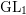$\text{GL}_{1}$

Published online by Cambridge University Press:  04 July 2019

Corresponding

## Abstract

We generalize our previous method on the subconvexity problem for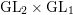$\text{GL}_{2}\times \text{GL}_{1}$ with cuspidal representations to Eisenstein series, and deduce a Burgess-like subconvex bound for Hecke characters, that is, the bound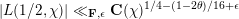$|L(1/2,\unicode[STIX]{x1D712})|\ll _{\mathbf{F},\unicode[STIX]{x1D716}}\mathbf{C}(\unicode[STIX]{x1D712})^{1/4-(1-2\unicode[STIX]{x1D703})/16+\unicode[STIX]{x1D716}}$ for varying Hecke characters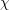$\unicode[STIX]{x1D712}$ over a number field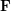$\mathbf{F}$ with analytic conductor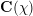$\mathbf{C}(\unicode[STIX]{x1D712})$ . As a main tool, we apply the extended theory of regularized integrals due to Zagier developed in a previous paper to obtain the relevant triple product formulas of Eisenstein series.

## MSC classification

Type
Research Article
Information
Compositio Mathematica , August 2019 , pp. 1457 - 1499

## Access options

Get access to the full version of this content by using one of the access options below.

## Footnotes

Research partially supported by SNF-grant 200021-125291 and DFG-SNF-grant 00021L_153647.

## References

Blomer, V. and Harcos, G., The spectral decomposition of shifted convolution sums , Duke Math. J. 144 (2008), 321339.CrossRefGoogle Scholar
Blomer, V. and Harcos, G., Twisted L-functions over number fields and Hilbert’s eleventh problem , Geom. Funct. Anal. 20 (2010), 152.CrossRefGoogle Scholar
Bump, D., Automorphic forms and representations, Cambridge Studies in Advanced Mathematics, vol. 55 (Cambridge University Press, Cambridge, 1998).Google Scholar
Burgess, D., On character sums and L-series II , Proc. Lond. Math. Soc. (3) 13 (1963), 524536.CrossRefGoogle Scholar
Conrey, J. B. and Iwaniec, H., The cubic moment of central values of automorphic L-functions , Ann. of Math. (2) 151 (2000), 11751216.CrossRefGoogle Scholar
Duke, W., Hyperbolic distribution problems and half-integral weight Maass forms , Invent. Math. 92 (1988), 7390.CrossRefGoogle Scholar
Duke, W., Friedlander, J. B. and Iwaniec, H., The subconvexity problem for Artin L-functions , Invent. Math. 149 (2002), 489577.CrossRefGoogle Scholar
Gelbart, S. S. and Jacquet, H., Forms of GL(2) from the analytic point of view , Proc. Sympos. Pure Math. 33 (1979), 213251.CrossRefGoogle Scholar
Heath-Brown, D., Hybrid bounds for Dirichlet L-functions , Invent. Math. 47 (1978), 149170.CrossRefGoogle Scholar
Heath-Brown, D., Hybrid bounds for Dirichlet L-functions II , Q. J. Math. (2) 31 (1980), 157167.Google Scholar
Huxley, M. and Watt, N., Hybrid bounds for Dirichlet’s L-function , Math. Proc. Cambridge Philos. Soc. 129 (2000), 385415.CrossRefGoogle Scholar
Jacquet, H., Integral representation of Whittaker functions , in Contributions to automorphic forms, geometry and number theory, eds Hida, H., Ramakrishnan, D. and Shahidi, F. (Johns Hopkins University Press, Baltimore, MD, 2004), 373419, ch. 15.Google Scholar
Michel, P., Analytic number theory and families of automorphic L-functions , in Automorphic forms and applications, IAS/Park City Mathematics Series, vol. 12 (American Mathematical Society, Providence, RI, 2007), 181295.Google Scholar
Michel, P. and Venkatesh, A., The subconvexity problem for GL2 , Publ. Math. Inst. Hautes Études Sci. 111 (2010), 171271.CrossRefGoogle Scholar
Milićević, D., Sub-Weyl subconvexity for Dirichlet L-functions to prime power moduli , Compositio Math. 152 (2016), 825875.CrossRefGoogle Scholar
Patterson, S., An introduction to the theory of the Riemann zeta-function, Cambridge Studies in Advanced Mathematics, vol. 14 (Cambridge University Press, Cambridge, 1988).CrossRefGoogle Scholar
Petrow, I. and Young, M. P., A generalized cubic moment and the Petersson formula for newforms , Math. Ann. 373 (2018), 287353.CrossRefGoogle Scholar
Rudin, W., Real and complex analysis, third edition (Tata McGraw-Hill, New Delhi, 1986).Google Scholar
Sarnak, P., Estimates for Ranking–Selberg L-functions and quantum unique ergodicity , J. Funct. Anal. 184 (2001), 419453.CrossRefGoogle Scholar
Soehne, P., An upper bound for Hecke zeta-functions with Groessencharacters , J. Number Theory 66 (1997), 225250, NT972167.CrossRefGoogle Scholar
Weyl, H., Zur Abschätzung von 𝜁(1 + ti) , Math. Z. 10 (1921), 88101.CrossRefGoogle Scholar
Wu, H., Burgess-like subconvex bounds for GL2 × GL1 , Geom. Funct. Anal. 24 (2014), 9681036.CrossRefGoogle Scholar
Wu, H., Explicit Burgess-like subconvex bounds for$\text{GL}_{2}\times \text{GL}_{1}$ , Preprint (2017), arXiv:1712.04365.Google Scholar
Wu, H., A note on spectral analysis in automorphic representation theory for GL2 : I , Int. J. Number Theory 13 (2017), 27172750.CrossRefGoogle Scholar
Wu, H., Deducing Selberg trace formula via Rankin–Selberg method for$\text{GL}_{2}$ . Trans. Amer. Math. Soc., to appear. Preprint (2018), arXiv:1810.09437.Google Scholar
Young, M. P., Weyl-type hybrid subconvexity bounds for twisted L-functions and Heegner points on shrinking sets , J. Eur. Math. Soc. (JEMS) 19 (2017), 15451576.CrossRefGoogle Scholar
Zagier, D., The Rankin–Selberg method for automorphic functions which are not of rapid decay , J. Fac. Sci. 28 (1982), 415438.Google Scholar

### Full text views

Full text views reflects PDF downloads, PDFs sent to Google Drive, Dropbox and Kindle and HTML full text views.

Total number of HTML views: 10
Total number of PDF views: 103 *
View data table for this chart

* Views captured on Cambridge Core between 04th July 2019 - 24th January 2021. This data will be updated every 24 hours.

Hostname: page-component-76cb886bbf-r88h9 Total loading time: 0.759 Render date: 2021-01-24T01:09:54.983Z Query parameters: { "hasAccess": "0", "openAccess": "0", "isLogged": "0", "lang": "en" } Feature Flags: { "shouldUseShareProductTool": true, "shouldUseHypothesis": true, "isUnsiloEnabled": true, "metricsAbstractViews": false, "figures": false, "newCiteModal": false }

# Send article to Kindle

Note you can select to send to either the @free.kindle.com or @kindle.com variations. ‘@free.kindle.com’ emails are free but can only be sent to your device when it is connected to wi-fi. ‘@kindle.com’ emails can be delivered even when you are not connected to wi-fi, but note that service fees apply.

Find out more about the Kindle Personal Document Service.

Burgess-like subconvexity for$\text{GL}_{1}$Available formats × # Send article to Dropbox To send this article to your Dropbox account, please select one or more formats and confirm that you agree to abide by our usage policies. If this is the first time you use this feature, you will be asked to authorise Cambridge Core to connect with your <service> account. Find out more about sending content to Dropbox. Burgess-like subconvexity for$\text{GL}_{1}$
Available formats
×

# Send article to Google Drive

To send this article to your Google Drive account, please select one or more formats and confirm that you agree to abide by our usage policies. If this is the first time you use this feature, you will be asked to authorise Cambridge Core to connect with your <service> account. Find out more about sending content to Google Drive.

Burgess-like subconvexity for$\text{GL}_{1}\$
Available formats
×
×